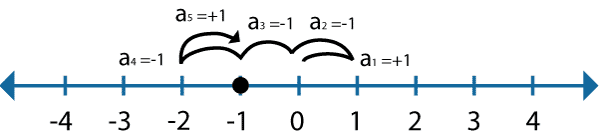Random Walks
The Mathematics in 1 Dimension

What is a random walk?

A random walk is the process by which randomly-moving objects wander away from where they started. The video below shows 7 black dots that start in one place randomly walking away. We will come back to this video when we know a little more about random walks.How can we describe this mathematically?

The simplest random walk to understand is a 1-dimensional walk. Suppose that the black dot below is sitting on a number line. The black dot starts in the center.Then, it takes a step, either forward or backward, with equal probability. It keeps taking steps either forward or backward each time. Let's call the 1st step a1, the second step a2, the third step a3 and so on. Each "a" is either equal to +1 (if the step is forward) or -1 (if the step is backward). The picture below shows a black dot that has taken 5 steps and ended up at -1 on the number line.Suppose we put the black dot at 0 and then let it take N steps (where N is any number). Now we want to know how far the black dot travels after it has taken N steps. Of course the distance traveled after N steps will vary each time we repeat the experiment, so what we want to know is, if we repeat the experiment many many times, how far the black dot will have traveled on average. Let's call the distance that the black dot has traveled "d". Keep in mind that d can either be positive or negative, depending on whether the black dot ends up to the right or left of 0. We know that for any one time that we repeat the experiment,

d = a1 + a2 + a3 + ... + aN

Now we use the notation <d> to mean "the average of d if we repeated the experiments many times":

<d> = <(a1 + a2 + a3 + ... + aN)> = <a1> + <a2> + <a3> + ... + <aN>

But <a1>=0, because if we repeated the experiment many many times, and a1 has an equal probability of being -1 or +1, we expect the average of a1 to be 0. So then,

<d> = <a1> + <a2> + <a3> + ... + <aN> = 0 + 0 + 0 + ... + 0 = 0

This isn't too surprising if you think about it. After all, <d> is the average location of the black dot after N steps, and since the dot is equally likely to move forward or backwards, we expect d to be 0, on average.

Well that was useless!

That exercise didn't tell us anything about how far the black dot gets after N steps! We are going to have to be a little more clever if we want to find out any kind of useful information.

Here's an idea... Even though d can be positive or negative, d2 is always positive, so it can't average out to 0. What if we found the average value of d2?

<d2> = <(a1 + a2 + a3 + ... + aN)2> = <(a1 + a2 + a3 + ... + aN) (a1 + a2 + a3 + ... + aN)>

= (<a12> + <a22> + <a32> + ... + <aN2>) + 2 (<a1a2> + <a1a3> + ... <a1aN> + <a2a3> + ... <a2aN> + ...)

First let's think about what <a12> is equal to. Well a1 can either be +1 or -1. Either way, a12 =1. Then on average a12 is 1 and <a12> = 1. The same applies to <a22>, <a32>, and all the way up to <aN2>.

Now let's think about what <a1a2> is equal to. There are 4 possible combinations of a1 and a2, each with equal probability:

 a1 a2 a1a2 1 1 1 1 -1 -1 -1 1 -1 -1 -1 1

Since a1a2 is equally likely to be +1 or -1, <a1a2> = 0. The same is true of all of <a1a3>, <a1aN>, <a2a3>, <a2aN> and all of the other terms containing two different steps. Then:

<d2> = (<a12> + <a22> + <a32> + ... + <aN2>) + 2 (<a1a2> + <a1a3> + ... <a1aN> + <a2a3> + ... <a2aN> + ...)

= (1 + 1 + 1 + ... +1) + 2 (0 + 0 + ... + 0 + 0 + ...) = N

Now we're getting somewhere! The average of the square of the distance is equal to N. If we take the square root of this equation, we realize that:

sqrt(<d2>)=sqrt(N)

Since sqrt(<d2>) is something like the average positive distance away from 0 after N steps (technically, it's called the "root-mean-squared" distance), we expect that after N steps, the black dot will be roughly sqrt(N) steps away from where it started. So for 25 steps, we expect the black dot to have moved roughly 5 total spaces from 0 in either direction. Of course sometimes it will move more and sometimes fewer total spaces, but 5 is roughly what we might expect.